#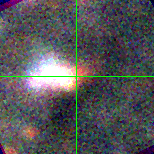M33-6 (J013328.75+304026.3)

## Target Notes### Classification: -

Khan et al. 2012. LGGS uncertain. Two stars one magnitude fainter within two arc seconds. Sometimes confused with other bright stars within several arc seconds (marked "X" in those cases) but usually not present.

## Plots of Brightness and Color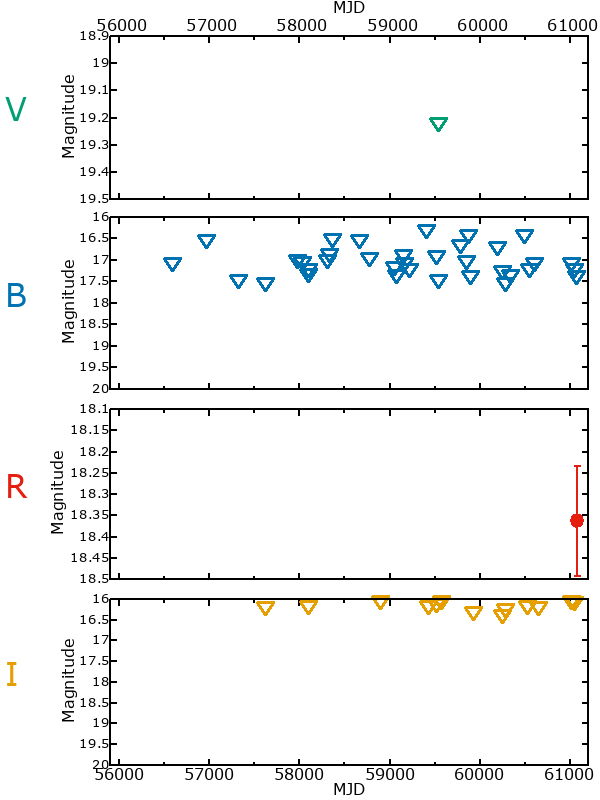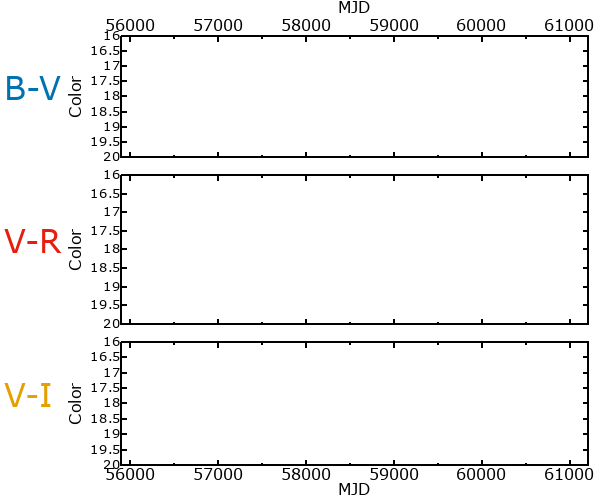## Variability

There are not yet enough observations (fewer than three epochs) of this star to reliably determine if it could be variable or not

### Correlation factors between bands

If the brightness variation is true variability then there should be a correlation between brightness changes recorded in different filter bands. The following table are the R-squared linear correlation factors calculated for the pairs of bands. The number in parentheses next to the R-squared value is the number of elements used to calculate the correlation factor. The number in square brackets next to the band is the range of magnitudes measured.

V [0.00 mag]B [0.00 mag]R [0.00 mag]
B [0.00 mag]-.-- (0)
R [0.00 mag]-.-- (0)-.-- (0)
I [0.00 mag]-.-- (0)-.-- (0) -.-- (1)

### Magnitude-Magnitude Plots

The plots below are provided to check the values in the table above and also to show any non-linear correlations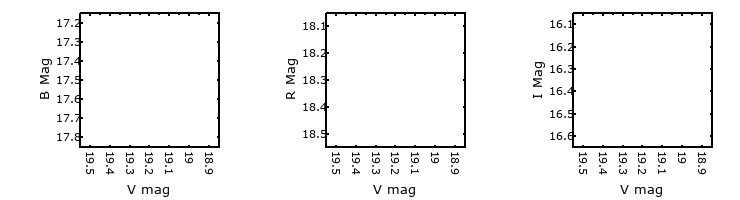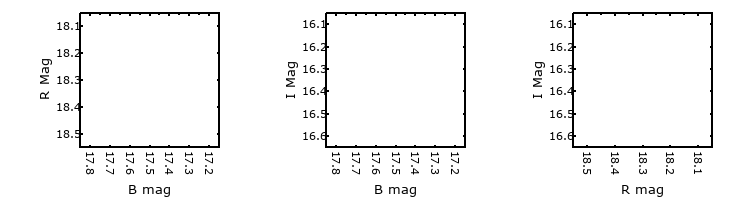### I & R Statistics

Welch & Stetson (1993, AJ, 105, 1813) present a method for varible star detection in CCD images from automated surveys. Data from two bands are compared and two statistics are calculated: R and I.

The I-statistic has an expectation value of zero for stars that are NOT variable. A larger non-zero I-value indicates a higher likelihood that the star is variable. The R statistic indicates how the color of the star changes as the variable grows brighter with R = 1.0 being no change in color.

R and I values have only been calculated if there are more than four (4) matched measurements of brightness in each band.
V
(I|R)
B
(I|R)
R
(I|R)
B
(I|R)
--- | ---
R
(I|R)
--- | ------ | ---
I
(I|R)
--- | ------ | --- --- | ----